# Electronics and Communication Engineering - Materials and Components

### Exercise :: Materials and Components - Section 3

21.

The dielectric constant of ferroelectric materials can be expressed by the equation

 A.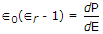B.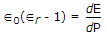C.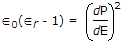D.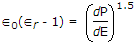Explanation:

No answer description available for this question. Let us discuss.

22.

A liquid has N permanent dipoles μp per unit volume and has been subjected to dc field for a long time such that orientation polarization is P0. At t = 0, the field is suddenly removed, then polarization

 A. will remain constant at P0 B. will become zero at t = 0 C. will delay to zero with a time constant D. either b or c

Explanation:

No answer description available for this question. Let us discuss.

23.

Assertion (A): Orbital magnetic moment of an electron in an atom is of the order of 1 Bohr magneton.

Reason (R): 1 Bohr magneton = 9.27 x 10-24 A/m2.

 A. Both A and R are true and R is correct explanation of A B. Both A and R are true but R is not correct explanation of A C. A is true but R is false D. A is false but R is true

Explanation:

No answer description available for this question. Let us discuss.

24.

As per Bohr's postulate, only those circular orbits are stable for which the angular momentum is equal to an integer times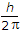.

 A. True B. False

Explanation:

It is a quantum condition postulated by Bohr.

25.

Inductors used at V.H.F. are made using

 A. air core B. cast iron cored C. sheet steel cored D. thin film technique